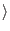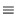Next: The Quantum Memory Register Up: The Quantum Computer Previous: Superposition and Eigenstates   Contents

## The Quantum qubit

Back to our qubit, our spin-1/2 particle. Now that we know that while it can only be measured to have a spin of +1/2 or -1/2, it may in general be in a superposition of these states when we are not measuring it. We could refer to its state in the following manner.

Let x1 be the eigenstate corresponding to the spin +1/2 state, and let x0 be the eigenstate corresponding to the spin -1/2 state. Let X be the total state of our state vector, and let w1 and w0 be the complex numbers that weight the contribution of the base states to our total state, then in general:

| X= w0*| x0+ w1*| x1(w0, w1)

At this point it should be remembered that w0 and w1, the weighting factors of the base states are complex numbers, and that when the state of X is measured, we are guaranteed to find it to be in either the state:

0*| x0+ w1*| x1(0, w1)

or the state

w0*| x0+0*| x1(w0, 0)

This is analogous to a system you may be more familiar with, a vector with real weighting factors in the a two dimensional plane. Let the base states for this two dimensional plane be the unit vectors x, and y. In this case we know that the state of any vector V can be described in the following manner:

V = x0*x + y0*y(x0, y0)

Our state vector is a unit vector in a Hilbert space, which is similar to vector spaces you may be more familiar with, but it differs in that the lengths of the vectors are complex numbers. It is not necessary from a physics perspective for the state vector to be a unit vector (by which I mean it has a length of 1), but it makes for easier calculations further on, so I will assume from here on out that the state vector has length 1. This assumption does not invalidate any claims about the behavior of the state vector. To see how to convert a state vector of any length to length 1 see appendix A.

With all this in mind we have fully defined the basic building block of our quantum computer, the qubit. It is fundamentally different from our classical bit in that it can exist in any superposition of the 0 and 1 states when it is not being measured. (Barenco, Ekert, Sanpera, Machiavello)Next: The Quantum Memory Register Up: The Quantum Computer Previous: Superposition and Eigenstates   Contents
Matthew Hayward - Quantum Computing and Shor's Algorithm GitHub Repository